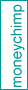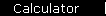Investment Performance Calculator

This calculator shows you how your portfolio is doing. Just give it your investment's beginning and ending balance for a given time period, and any additions and withdrawals (including dividends not kept in the account) along the way.

 Inputs
Starting Balance: \$
Ending Balance: \$
Months elapsed:
Total Withdrawals & Dividends: \$

 Results
Annualized Return Rate:   %

How Does it Work?

This calculator can only give you an estimate (total accuracy would require you to give the date and amount of each addition and withdrawal) but it's a respected estimate, using a formula recommended by The Four Pillars of Investing and The Motley Fool, and widely used by many others.

Let's take a couple of examples to see how the method works.

Example 1:   First, assume your account grows from \$1000 to \$1200 in one year, and that you don't make any additions or withdrawals during that time. That means your investments created \$200 of wealth, which is 20% of the \$1000 it had to work with - so the return rate must be twenty percent.

Example 2:   Now for a more complicated example. Again assume that your starting balance was \$1000 and your ending balance was \$1200, but that you made a \$50 addition at some point during the year. This time, your investments only created \$150 of new wealth. To turn that dollar figure into a percent, you have to decide "a percent of what?" - that is, how much money did the account have to work on during the year? Well, it had the initial \$1000 for the whole year, and the \$50 addition for some unknown portion of the year, so we'll use the estimate that it had the equivalent of \$1025 for the whole year (that is, the whole thousand, plus half of the fifty). Now the growth rate is

(\$150 growth) / (\$1025 estimated average principal) = 0.1463

or 14.63 percent.

See below (or one of the two links above) for a formula that you can write down or use in a spreadsheet. (You may have to do a little painful thinking to convince yourself that the formula really does come out of the logic described here.)

Investment Return Formula

The estimate used in Example 2 is that

\$1025  grew by  \$150

Equivalently (but more confusingly!)

\$1025  grew to  \$1175

or

( Bstart   + N / 2 )   grew to   ( Bend   - N / 2 )

where Bstart and Bend are the starting and ending balances, and N is the net additions minus withdrawals. Plugging these values into the return rate formula gives:

r   =   [ ( Bend   - N / 2 )   /   ( Bstart   + N / 2 ) ] 1/Y   -   1

where Y is the elapsed time, in years.

. . .

BUG FIX: The last formula shown was wrong before 9/26/2014. (The calculator was correct.) Apologies for the confusion.

 home  |  features  |  glossary  |  calculator  |  about us  |  books